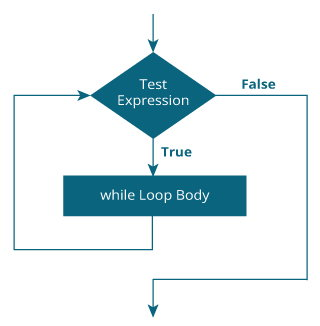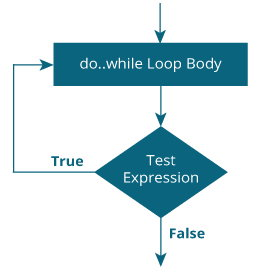Kotlin while and do...while Loop

# Kotlin while and do...while Loop

#### Loop is used in programming to repeat a specific block of code. In this article, you will learn to create while and do...while loops in Kotlin programming.

Loop is used in programming to repeat a specific block of code until certain condition is met (test expression is `false)`.

Loops are what makes computers interesting machines. Imagine you need to print a sentence 50 times on your screen. Well, you can do it by using print statement 50 times (without using loops). How about you need to print a sentence one million times? You need to use loops.

You will learn about two loops `while` and `do..while` in this article with the help of examples.

If you are familiar with while and do...while loops in Java, you are already familiar with these loops in Kotlin as well.

## Kotlin while Loop

The syntax of `while` loop is:

```while (testExpression) {
// codes inside body of while loop
}```

### How while loop works?

The test expression inside the parenthesis is a Boolean expression.

If the test expression is evaluated to `true`,

• statements inside the while loop are executed.
• then, the test expression is evaluated again.

This process goes on until the test expression is evaluated to `false`.

If the test expression is evaluated to `false`,

• while loop is terminated.

### Flowchart of while Loop### Example: Kotlin while Loop

```// Program to print line 5 times

```fun main(args: Array<String>) {

var i = 1

while (i <= 5) {
println("Line \$i")
++i
}
}``````

When you run the program, the output will be:

```Line 1
Line 2
Line 3
Line 4
Line 5```

Notice, `++i` statement inside the `while` loop. After 5 iterations, variable i will be incremented to 6. Then, the test expression `i <= 5` is evaluated to `false` and the loop terminates.

If the body of loop has only one statement, it's not necessary to use curly braces `{ }`

### Example: Compute sum of Natural Numbers

``````// Program to compute the sum of natural numbers from 1 to 100.
fun main(args: Array<String>) {

var sum = 0
var i = 100

while (i != 0) {
sum += i     // sum = sum + i;
--i
}
println("sum = \$sum")
}``````

When you run the program, the output will be:

`sum = 5050`

Here, the variable sum is initialized to 0 and i is initialized to 100. In each iteration of while loop, variable sum is assigned `sum + i`, and the value of i is decreased by 1 until i is equal to 0. For better visualization,

```1st iteration: sum = 0+100 = 100, i = 99
2nd iteration: sum = 100+99 = 199, i = 98
3rd iteration: sum = 199+98 = 297, i = 97
... .. ...
... .. ...
99th iteration: sum = 5047+2 = 5049, i = 1
100th iteration: sum = 5049+1 = 5050, i = 0 (then loop terminates)```

## Kotlin do...while Loop

The `do...while` loop is similar to `while` loop with one key difference. The body of `do...while` loop is executed once before the test expression is checked.

Its syntax is:

```do {
// codes inside body of do while loop
} while (testExpression);```

### How do...while loop works?

The codes inside the body of `do` construct is executed once (without checking the testExpression). Then, the test expression is checked.

If the test expression is evaluated to `true`, codes inside the body of the loop are executed, and test expression is evaluated again. This process goes on until the test expression is evaluated to `false`.

When the test expression is evaluated to `false`, `do..while` loop terminates.

### Flowchart of do...while Loop### Example: Kotlin do...while Loop

The program below calculates the sum of numbers entered by the user until user enters 0.

To take input from the user, `readline()` function is used. Recommended Reading: Kotlin Basic Input

``````fun main(args: Array<String>) {

var sum: Int = 0
var input: String

do {
print("Enter an integer: ")
sum += input.toInt()

} while (input != "0")

println("sum = \$sum")
}``````

When you run the program, the output will be something like:

```Enter an integer: 4
Enter an integer: 3
Enter an integer: 2
Enter an integer: -6
Enter an integer: 0
sum = 3
```